# Python图表绘制：matplotlib绘图库入门

+关注继续查看

matplotlib 是python最著名的绘图库，它提供了一整套和matlab相似的命令API，十分适合交互式地行制图。而且也可以方便地将它作为绘图控件，嵌入GUI应用程序中。

Matplotlib.pyplot快速绘图

matplotlib实际上是一套面向对象的绘图库，它所绘制的图表中的每个绘图元素，例如线条Line2D、文字Text、刻度等在内存中都有一个对象与之对应。

plt.plot()实际上会通过plt.gca()获得当前的Axes对象ax，然后再调用ax.plot()方法实现真正的绘图

matplotlib所绘制的图表的每个组成部分都和一个对象对应，我们可以通过调用这些对象的属性设置方法set_*()或者pyplot模块的属性设置函数setp()设置它们的属性值。

matplotlib将这些缺省配置保存在一个名为“matplotlibrc”的配置文件中，通过修改配置文件，我们可以修改图表的缺省样式。配置文件的读入可以使用rc_params()，它返回一个配置字典；在matplotlib模块载入时会调用rc_params()，并把得到的配置字典保存到rcParams变量中；matplotlib将使用rcParams字典中的配置进行绘图；用户可以直接修改此字典中的配置，所做的改变会反映到此后创建的绘图元素。

Matplotlib 里的常用类的包含关系为 Figure -> Axes -> (Line2D, Text, etc.)一个Figure对象可以包含多个子图(Axes)，在matplotlib中用Axes对象表示一个绘图区域，可以理解为子图。

subplot(numRows, numCols, plotNum)

subplot将整个绘图区域等分为numRows行* numCols列个子区域，然后按照从左到右，从上到下的顺序对每个子区域进行编号，左上的子区域的编号为1。如果numRows，numCols和plotNum这三个数都小于10的话，可以把它们缩写为一个整数，例如subplot(323)和subplot(3,2,3)是相同的。subplot在plotNum指定的区域中创建一个轴对象。如果新创建的轴和之前创建的轴重叠的话，之前的轴将被删除。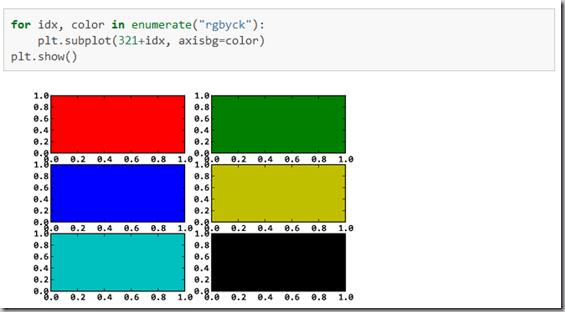subplot()返回它所创建的Axes对象，我们可以将它用变量保存起来，然后用sca()交替让它们成为当前Axes对象，并调用plot()在其中绘图。

import numpy as np
import matplotlib.pyplot as plt

plt.figure(1) # 创建图表1
plt.figure(2) # 创建图表2
ax1 = plt.subplot(211) # 在图表2中创建子图1
ax2 = plt.subplot(212) # 在图表2中创建子图2

x = np.linspace(0, 3, 100)
for i in xrange(5):
    plt.figure(1)  # # 选择图表1
    plt.plot(x, np.exp(i*x/3))
    plt.sca(ax1)   # # 选择图表2的子图1
    plt.plot(x, np.sin(i*x))
    plt.sca(ax2)  # 选择图表2的子图2
    plt.plot(x, np.cos(i*x))

plt.show()matplotlib的缺省配置文件中所使用的字体无法正确显示中文。为了让图表能正确显示中文，可以有几种解决方案。

1. 在程序中直接指定字体。

2. 在程序开头修改配置字典rcParams。

3. 修改配置文件。

matplotlib API包含有三层，Artist层处理所有的高层结构，例如处理图表、文字和曲线等的绘制和布局。通常我们只和Artist打交道，而不需要关心底层的绘制细节。

• 创建Figure对象

• 用Figure对象创建一个或者多个Axes或者Subplot对象

• 调用Axies等对象的方法创建各种简单类型的Artists

import matplotlib.pyplot as plt



X1 = range(0, 50) Y1 = [num**2 for num in X1] # y = x^2 X2 = [0, 1] Y2 = [0, 1] # y = x



Fig = plt.figure(figsize=(8,4)) # Create a figure' instance Ax = Fig.add_subplot(111) # Create a axes' instance in the figure Ax.plot(X1, Y1, X2, Y2) # Create a Line2D instance in the axes



Fig.show() Fig.savefig("test.pdf")

《Python科学计算》(Numpy视频matplotlib-绘制精美的图表(快速绘图)(面向对象绘图)（深入浅出适合系统学习）

Matplotlib.pylab快速绘图

matplotlib还提供了一个名为pylab的模块，其中包括了许多NumPy和pyplot模块中常用的函数，方便用户快速进行计算和绘图，十分适合在IPython交互式环境中使用。这里使用下面的方式载入pylab模块：

>>> import pylab as pl

1 安装numpy和matplotlib

>>> import numpy
>>> numpy.__version__

>>> import matplotlib
>>> matplotlib.__version__

2 两种常用图类型：Line and scatter plots(使用plot()命令), histogram(使用hist()命令)

2.1 折线图&散点图 Line and scatter plots

2.1.1 折线图 Line plots(关联一组x和y值的直线)

import numpy as np
import pylab as pl

x = [1, 2, 3, 4, 5]# Make an array of x values
y = [1, 4, 9, 16, 25]# Make an array of y values for each x value

pl.plot(x, y)# use pylab to plot x and y
pl.show()# show the plot on the screen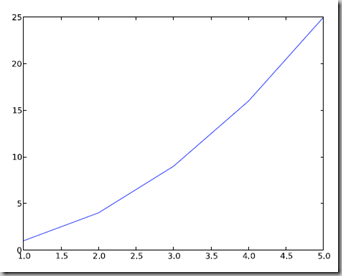2.1.2 散点图 Scatter plots

2.2  美化 Making things look pretty

2.2.1 线条颜色 Changing the line color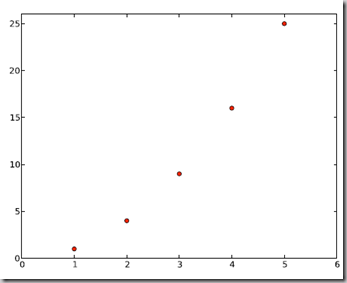2.2.2 线条样式 Changing the line style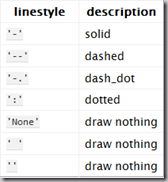2.2.3 marker样式 Changing the marker style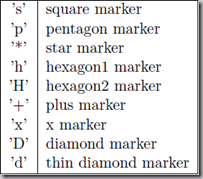2.2.4 图和轴标题以及轴坐标限度 Plot and axis titles and limits

import numpy as np
import pylab as pl

x = [1, 2, 3, 4, 5]# Make an array of x values
y = [1, 4, 9, 16, 25]# Make an array of y values for each x value
pl.plot(x, y)# use pylab to plot x and y

pl.title(’Plot of y vs. x’)# give plot a title
pl.xlabel(’x axis’)# make axis labels
pl.ylabel(’y axis’)

pl.xlim(0.0, 7.0)# set axis limits
pl.ylim(0.0, 30.)

pl.show()# show the plot on the screen2.2.5 在一个坐标系上绘制多个图 Plotting more than one plot on the same set of axes

import numpy as np
import pylab as pl

x1 = [1, 2, 3, 4, 5]# Make x, y arrays for each graph
y1 = [1, 4, 9, 16, 25]
x2 = [1, 2, 4, 6, 8]
y2 = [2, 4, 8, 12, 16]

pl.plot(x1, y1, ’r’)# use pylab to plot x and y
pl.plot(x2, y2, ’g’)

pl.title(’Plot of y vs. x’)# give plot a title
pl.xlabel(’x axis’)# make axis labels
pl.ylabel(’y axis’)


pl.xlim(0.0, 9.0)# set axis limits
pl.ylim(0.0, 30.)


pl.show()# show the plot on the screen2.2.6  图例 Figure legends

pl.legend((plot1, plot2), (’label1, label2’), 'best’, numpoints=1)

import numpy as np
import pylab as pl

x1 = [1, 2, 3, 4, 5]# Make x, y arrays for each graph
y1 = [1, 4, 9, 16, 25]
x2 = [1, 2, 4, 6, 8]
y2 = [2, 4, 8, 12, 16]

plot1 = pl.plot(x1, y1, ’r’)# use pylab to plot x and y : Give your plots names
plot2 = pl.plot(x2, y2, ’go’)

pl.title(’Plot of y vs. x’)# give plot a title
pl.xlabel(’x axis’)# make axis labels
pl.ylabel(’y axis’)


pl.xlim(0.0, 9.0)# set axis limits
pl.ylim(0.0, 30.)


pl.legend([plot1, plot2], (’red line’, ’green circles’), ’best’, numpoints=1)# make legend
pl.show()# show the plot on the screen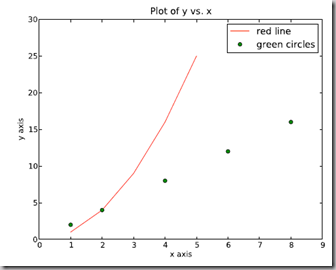2.3 直方图 Histograms

import numpy as np
import pylab as pl

# make an array of random numbers with a gaussian distribution with
# mean = 5.0
# rms = 3.0
# number of points = 1000
data = np.random.normal(5.0, 3.0, 1000)

# make a histogram of the data array
pl.hist(data)

# make plot labels
pl.xlabel(’data’)
pl.show()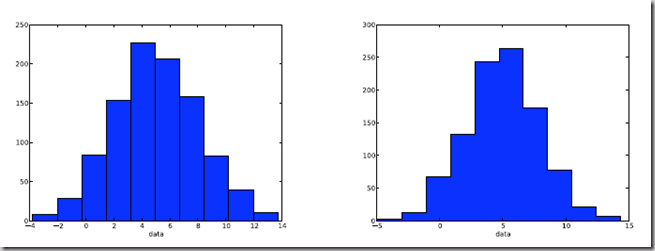2.3.1 自定义直方图bin宽度 Setting the width of the histogram bins manually

bins = np.arange(-5., 16., 1.) #浮点数版本的range
pl.hist(data, bins, histtype=’stepfilled’)3 同一画板上绘制多幅子图 Plotting more than one axis per canvas

fig1 = pl.figure(1)
pl.subplot(211)
subplot(211)把绘图区域等分为2行*1列共两个区域, 然后在区域1(上区域)中创建一个轴对象. pl.subplot(212)在区域2(下区域)创建一个轴对象。You can play around with plotting a variety of layouts. For example, Fig. 11 is created using the following commands:

f1 = pl.figure(1)
pl.subplot(221)
pl.subplot(222)
pl.subplot(212)4 绘制文件中的数据Plotting data contained in files

4.1 从Ascii文件中读取数据 Reading data from ascii files

**********************************************

# fakedata.txt
0 0
1 1
2 4
3 9
4 16
5 25
6 36
7 49
8 64
9 81
0 0
1 1
2 4
3 9
4 16
5 25
6 36
7 49
8 64
9 81

**********************************************

import numpy as np
import pylab as pl

# Use numpy to load the data contained in the file
# ’fakedata.txt’ into a 2-D array called data
data = np.loadtxt(’fakedata.txt’)

# plot the first column as x, and second column as y
pl.plot(data[:,0], data[:,1], ’ro’)
pl.xlabel(’x’)
pl.ylabel(’y’)
pl.xlim(0.0, 10.)
pl.show()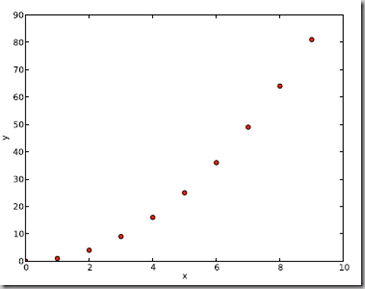4.2 写入数据到文件 Writing data to a text file

import numpy as np
# Let’s make 2 arrays (x, y) which we will write to a file
# x is an array containing numbers 0 to 10, with intervals of 1
x = np.arange(0.0, 10., 1.)
# y is an array containing the values in x, squared
y = x*x
print ’x = ’, x
print ’y = ’, y

# Now open a file to write the data to
# ’w’ means open for ’writing’
file = open(’testdata.txt’, ’w’)
# loop over each line you want to write to file
for i in range(len(x)):
    # make a string for each line you want to write
    # ’\t’ means ’tab’
    # ’\n’ means ’newline’
    # ’str()’ means you are converting the quantity in brackets to a string type
    txt = str(x[i]) + ’\t’ + str(y[i]) + ’ \n’
    # write the txt to the file
    file.write(txt)
# Close your file
file.close()

Matlplotlib对LaTeX有一定的支持，如果记得使用raw字符串语法会很自然：

xlabel(r"x2y4")

Here is a simple example:

# plain text
plt.title('alpha > beta')

produces “alpha > beta”.

Whereas this:produces "".

import matplotlib.pyplot as plt

x = arange(1,1000,1)
r = -2
c = 5
y = [5*(a**r) for a in x]

fig = plt.figure()

ax.loglog(x,y,label = r"y=12σ21,c=5,σ1=2")
ax.legend()
ax.set_xlabel(r"x")
ax.set_ylabel(r"y")

plt.plot(x,y,label="sin(x)",color="red",linewidth=2)
plt.plot(x,z,"b--",label="cos(x2)")

plot函数的调用方式很灵活，第一句将x,y数组传递给plot之后，用关键字参数指定各种属性：

• label : 给所绘制的曲线一个名字，此名字在图示(legend)中显示。只要在字符串前后添加"\$"符号，matplotlib就会使用其内嵌的latex引擎绘制的数学公式。

• color : 指定曲线的颜色

• linewidth : 指定曲线的宽度

Writing mathematical expressions

Text rendering With LaTeX

• matplotlib.rcParams属性字典

• 想要它正常工作，在matplotlibrc配置文件中需要设置text.markup = "tex"。

• 如果你希望图表中所有的文字（包括坐标轴刻度标记）都是LaTeX'd,需要在matplotlibrc中设置text.usetex = True。如果你使用LaTeX撰写论文，那么这一点对于使图表和论文中其余部分保持一致是很有用的。

• 在matplotlib中使用中文字符串时记住要用unicode格式，例如：u''测试中文显示''

matplotlib使用小结

LaTeX科技排版

ax.semilogx(x,y) #x轴为对数坐标轴

ax.semilogy(x,y) #y轴为对数坐标轴

ax.loglog(x,y) #双对数坐标轴

1、找到了matplotlibrc设置文件,是个文本文件,随便找个编辑器打开它,找到font.sans-serif一行,将后边直接改成一个nothing；（把 “：”后的“#......”都去掉）

2、找到verbose.level一行,把默认的silent改成debug.

1.       # coding = utf-8

2.        from pylab import *

3.        x = [2,4,6,8,10]

4.        y = [1224,838,632,626,624]

5.        xlabel(u"text for x轴")

6.        ylabel(u"text for y轴")

7.        title(u"x轴和Y轴对应关系")

8.        plot(x,y)

9.        savefig('test')

10.    show()Python中，如何使用ImageDraw在Image上绘制粗线？
Python中，如何使用ImageDraw在Image上绘制粗线？
60 083 0python--使用cnmaps绘制省界地图（快速上手，简单有效）
cnmaps是一个致力于让中国地图的获取和使用更丝滑的python扩展包。
527 0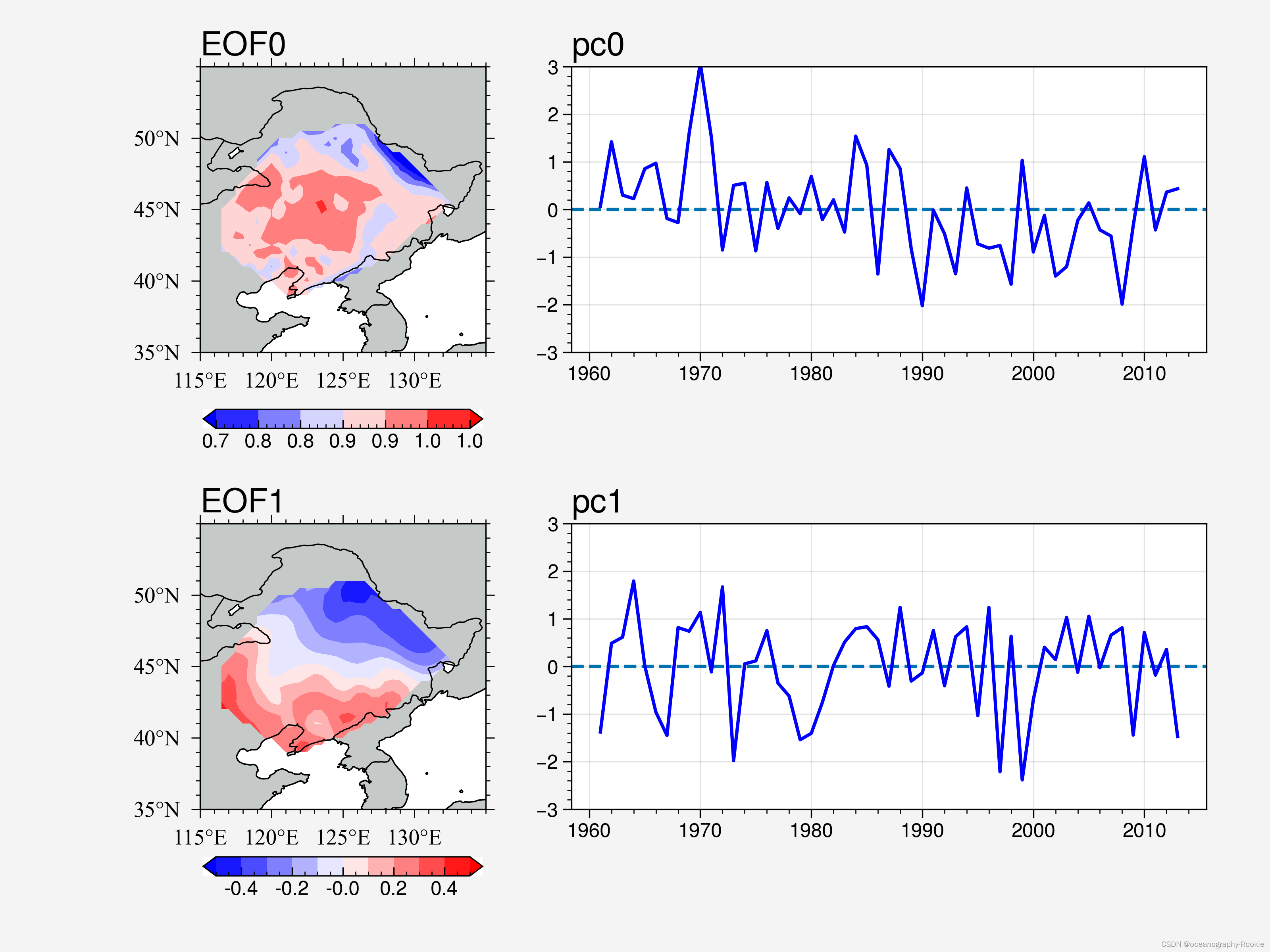python--对站点数据做EOF并做插值绘制填色图

111 0python读取excel数据、转换度分为度，绘制填色图
python读取excel数据、转换度分为度，绘制填色图
72 0python--循环绘制ERA5风场的空间分布图

124 0python绘图--由逐日风场数据计算月平均风场数据并绘制二维填色图
python绘图--由逐日风场数据计算月平均风场数据并绘制二维填色图
212 0

Python基础快速入门实战教程377918